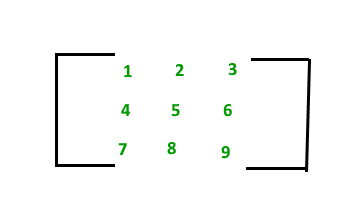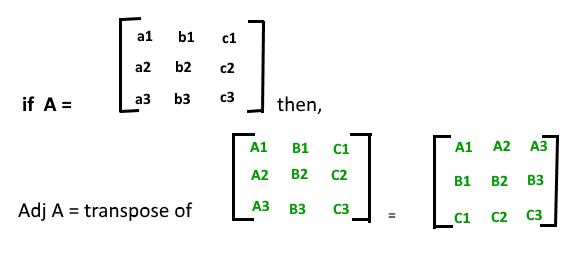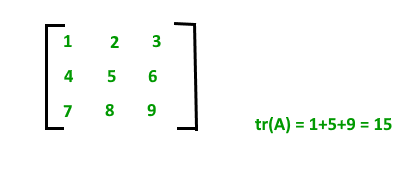# What is Engineering Mathematics?

Engineering Mathematics is the art of applying mathematics to complex real-world problems. It combines mathematical theory, practical engineering and scientific computing to address today’s technological challenges or

Engineering mathematics is a branch of applied mathematics concerning mathematical methods and techniques that are typically used in engineering and industry. Along with fields like engineering physics and engineering geology, both of which may belong in the wider category engineering science, engineering mathematics is an interdisciplinary subject motivated by engineers’ needs both for practical, theoretical and other considerations outwith their specialization, and to deal with constraints to be effective in their work.

Engineering Mathematics is a creative and exciting discipline, spanning traditional boundaries. Engineering mathematicians can be found in an extraordinarily wide range of careers, from designing next generation Formula One cars to working at the cutting edge of robotics, from running their own business creating new autonomous vehicles to developing innovative indices for leading global financial institutions.

# Linear Algebra

### Mathematics | Matrix Introduction

A matrix represents a collection of numbers arranged in an order of rows and columns. It is necessary to enclose the elements of a matrix in parentheses or brackets.
A matrix with 9 elements is shown below.This Matrix [M] has 3 rows and 3 columns. Each element of matrix [M] can be referred to by its row and column number. For example, a23=6

Order of a Matrix :
The order of a matrix is defined in terms of its number of rows and columns.
Order of a matrix = No. of rows ×No. of columns
Therefore Matrix [M] is a matrix of order 3 × 3.

Transpose of a Matrix :
The transpose [M]T of an m x n matrix [M] is the n x m matrix obtained by interchanging the rows and columns of [M].
if A= [aij] mxn , then AT = [bij] nxm where bij = aji

Properties of transpose of a matrix:

• (AT)T = A
• (A+B)T = AT + BT
• (AB)T = BTAT

Singular and Nonsingular Matrix:

1. Singular Matrix: A square matrix is said to be singular matrix if its determinant is zero i.e. |A|=0
2. Nonsingular Matrix: A square matrix is said to be non-singular matrix if its determinant is non-zero.

Properties of Matrix addition and multiplication:

1. A+B = B+A (Commutative)
2. (A+B)+C = A+ (B+C) (Associative)
3. AB ≠ BA (Not Commutative)
4. (AB) C = A (BC) (Associative)
5. A (B+C) = AB+AC (Distributive)

Square Matrix: A square Matrix has as many rows as it has columns. i.e. no of rows = no of columns.
Symmetric matrix: A square matrix is said to be symmetric if the transpose of original matrix is equal to its original matrix. i.e. (AT) = A.
Skew-symmetric: A skew-symmetric (or antisymmetric or antimetric) matrix is a square matrix whose transpose equals its negative.i.e. (AT) = -A.

Diagonal Matrix:A diagonal matrix is a matrix in which the entries outside the main diagonal are all zero. The term usually refers to square matrices.
Identity Matrix:A square matrix in which all the elements of the principal diagonal are ones and all other elements are zeros.Identity matrix is denoted as I.
Orthogonal Matrix: A matrix is said to be orthogonal if AAT = ATA = I
Idemponent Matrix: A matrix is said to be idempotent if A2 = A
Involutary Matrix: A matrix is said to be Involuntary if A2 = I.

Note: Every Square Matrix can uniquely be expressed as the sum of a symmetric matrix and skew-symmetric matrix. A = 1/2 (AT + A) + 1/2 (A – AT).Inverse of a square matrix:Here |A| should not be equal to zero, means matrix A should be non-singular.

Properties of inverse:

1. (A-1)-1 = A
2. (AB)-1 = B-1A-1
3. only a non singular square matrix can have an inverse.

Where should we use the inverse matrix?

If you have a set of simultaneous equations:

7x + 2y + z = 21
3y – z = 5
-3x + 4y – 2x = -1

As we know when AX = B, then X = A-1B so we calculate the inverse of A and by multiplying it B, we can get the values of x, y, and z.

Trace of a matrix: trace of a matrix is denoted as tr(A) which is used only for square matrix and equals the sum of the diagonal elements of the matrix. Remember trace of a matrix is also equal to sum of eigen value of the matrix. For example:## STEM Elearning

We at FAWE have built this platform to aid learners, trainers and mentors get practical help with content, an interactive platform and tools to power their teaching and learning of STEM subjects, more